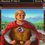# An interesting exponent.

A few days ago, my Calculus professor discussed exponential functions in general. Apart from the easy stuff, he actually asked us something that caught my attention and yet puzzled me greatly. The problem at hand was the following:

Approximate $b^{\sqrt{2}}$, where $b \in (-\infty,\infty)$?

Furthermore, he mentioned that this can be solved using techniques for different representation of real numbers. This is something I have never come across thus far, and would love it if someone gave me a more thorough explanation if possible.

Needless to say, I would also like to see this problem generalized a bit.

Therefore, my questions are:

Given that $x=b^{\sqrt{n}}$, where $b$ and $n$ are real numbers, is there a valid way of finding $x$? If so, how does one do it and can you provide a valid proof?

Thank you very much in advance!

Note: Feel free to point at any literature that can answer my questions.Note by Ivan Sekovanić
7 years, 5 months ago

This discussion board is a place to discuss our Daily Challenges and the math and science related to those challenges. Explanations are more than just a solution — they should explain the steps and thinking strategies that you used to obtain the solution. Comments should further the discussion of math and science.

When posting on Brilliant:

• Use the emojis to react to an explanation, whether you're congratulating a job well done , or just really confused .
• Ask specific questions about the challenge or the steps in somebody's explanation. Well-posed questions can add a lot to the discussion, but posting "I don't understand!" doesn't help anyone.
• Try to contribute something new to the discussion, whether it is an extension, generalization or other idea related to the challenge.

MarkdownAppears as
*italics* or _italics_ italics
**bold** or __bold__ bold
- bulleted- list
• bulleted
• list
1. numbered2. list
1. numbered
2. list
Note: you must add a full line of space before and after lists for them to show up correctly
paragraph 1paragraph 2

paragraph 1

paragraph 2

[example link](https://brilliant.org)example link
> This is a quote
This is a quote
    # I indented these lines
# 4 spaces, and now they show
# up as a code block.

print "hello world"
# I indented these lines
# 4 spaces, and now they show
# up as a code block.

print "hello world"
MathAppears as
Remember to wrap math in $$ ... $$ or $ ... $ to ensure proper formatting.
2 \times 3 $2 \times 3$
2^{34} $2^{34}$
a_{i-1} $a_{i-1}$
\frac{2}{3} $\frac{2}{3}$
\sqrt{2} $\sqrt{2}$
\sum_{i=1}^3 $\sum_{i=1}^3$
\sin \theta $\sin \theta$
\boxed{123} $\boxed{123}$

Sort by:

Let's just consider the $n = 2$ and $b > 0$ case: suppose that the function $f(x) = b^x$ is uniformly continuous when $b > 0$, then we can represent $\sqrt{2} = \lim x_k$ as the limit of some rational cauchy sequence. By uniform continuity, we can also claim that b^\sqrt{2} = f\left(\lim_k x_k\right)= \lim_k f(x_k) this means that we can construct a convergent sequence to b^\sqrt{2} by taking rational exponents, so for example, if we have nice technique/method of computing rational exponents, then we can compute 3^\sqrt{2} by computing $3^1, 3^{1.4}, 3^{1.41}, 3^{1.414}, 3^{1.4142},\dots$

From a computational point of view, the selection of the sequence matters because sequences that converges quicker will yield a better approximation. In the above, we can bound the error of $|x_k - \sqrt{2}| \le 10^{-k}$, so the relative error of the $k^{th}$ approximation is bounded above by $1 - b^{10^{-k}}$. Doing a bit of quick and dirty work (using a first order approximation of $b^x = x\log b + O(x^2)$, and let $\delta_k$ denote the relative error using the $k^{th}$ approximation, then for errors of the form $1 - b^{f(k)}$ $\delta_k \approx |f(k)\log b|$

For the sequence that adds in an extra decimal term each time, we can arbitrarily guarantee $m$ digits of accuracy in decimal point by allowing $10^{-m} \approx 10^{-k} \log(b) \implies k \approx m + \log \log b$

More interestingly, the following rational sequence approximating $\sqrt{2} = \lim_k x_k$ where the sequence is described by the recurrence $x_{k+1} = \frac{x_k^2 + 2}{2x_k} ~~~~ x_1 = \frac{3}{2}$ converges quite rapidly. In fact, within the region $x_k \in (1,2)$, it can be shown that $|\sqrt{2} - x_k| \le \frac{1}{2} \left(\frac{\sqrt{2} - 1}{2}\right)^{2^k}$ so here, to get m digits in decimal, you would only need to compute the $k = \log_2(m) - \log_{2}\log_{10}\left(\frac{2}{\sqrt{2}-1}\right)$, which is super quick!

- 7 years, 5 months ago

This is truly astounding and answers my question completely. Thank you very much!

- 7 years, 5 months ago

We can write $b^{\sqrt{2}} = e^{\sqrt{2} \log b }$, from which it follows that if $b > 0$, the resulting function is well defined on the reals. When $b = 0$, then it is reasonable to interpret $0^{\sqrt{2}}$ as the limiting value of $f(x) = 0^x$ as $x \to \sqrt{2}$, which is of course $0$.

The interesting that happens is when $b < 0$. Can we formulate an axiomatically consistent interpretation of the function $g(b) = b^{\sqrt{2}}$ for $b < 0$? Can we do so for non-real $b$? The above suggests employing the complex logarithm: $\log z = \log |z| + i \arg z + 2 \pi i k, \quad k \in \mathbb Z,$ where $\arg z$ is the complex argument of $z$; i.e., the angle that $z$ makes with the positive real axis in the complex plane.

With this in mind, we observe that for general complex $b$, the expression $b^{\sqrt{2}}$ is a set of complex numbers. We can choose a particular branch for this multivalued function to make it well-defined, but the result will contain a branch cut, across which the value of the function will be discontinuous.

- 7 years, 5 months ago

Amazing! Thank you very much!

- 7 years, 5 months ago

god bless

- 6 years, 6 months ago